# Total Dominator Chromatic Number of a Hypercube (Solved)

 Importance: Medium ✭✭
 Subject: Graph Theory » Coloring » » Vertex coloring
 Keywords: Total dominator chromatic number, hypercube.
 Posted by: Adel P. Kazemi on: October 6th, 2013
 Solved by: See comment.
Conjecture   For any integer,.

Here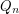denotes the-dimensional hypercube, i.e. the graph with vertex set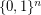and two vertices adjacent if they differ in exactly one coordinate. A total dominator coloring of a graph, briefly TDC, is a proper coloring ofin which each vertex of the graph is adjacent to every vertex of some color class. The total dominator chromatic number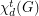ofis the minimum number of color classes in a TDC in(see [Kaz1]). A total dominating set of a graphis a setof vertices ofsuch that every vertex has at least one neighbor in". The total domination numberofis the cardinality of a minimum total dominating set.

The following theorems are proved in [Kaz2].

Theorem   For any integer,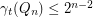.
Theorem   For any integer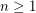,.
Theorem   1. If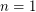, then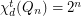.
2. If, then.
3. If, then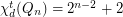.

## Bibliography

[Kaz1] Adel P. Kazemi, Total dominator chromatic number of a graph, http://arxiv.org/abs/1307.7486.

[Kaz2] Adel P. Kazemi, Total Dominator Coloring in Product Graphs, Utilitas Mathematica (2013), Accepted.

* indicates original appearance(s) of problem.

### False

Sinceit follows that for large enoughthe conjecture does not hold by the same token as written in the comment here http://www.openproblemgarden.org/op/total_domination_number_of_a_hypercube